Browse by Topic
Related Topics

# Seeing beyond a Theorem

Mathematics is a notoriously technical subject that prizes exactingly precise statements. The square of the hypotenuse of a right triangle is the sum of the squares of the legs, not the sum of their cubes, nor the difference of their squares. Such precision produces the clarity that makes the subject so powerful, but occasionally it comes at the cost of easy understanding. Indeed, more-complicated mathematical statements often sound bewildering upon first reading. Take the following theorem in plane geometry (deep breath…):

Let ABC be a triangle. Let DEF be parallel to AC with D on AB and E on BC. Let FGH be parallel to AB with G on BC and H on AC. Let,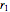,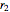and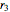be the radii of the incircles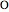,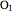,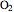and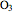of the triangles ABC, DBE, EFG and HGC, respectively. If F is outside of ABC, then.

Got it? Many theorems of mathematics, including this one, are easier to communicate by picture than by words. Here’s the scenario described in the theorem (images in this post are produced by slightly modified versions of the code for the Demonstration “The Radii of Four Incircles,” which is one of nearly 200 Demonstrations about theorems in plane geometry written by Jay Warendorff for the Wolfram Demonstrations Project):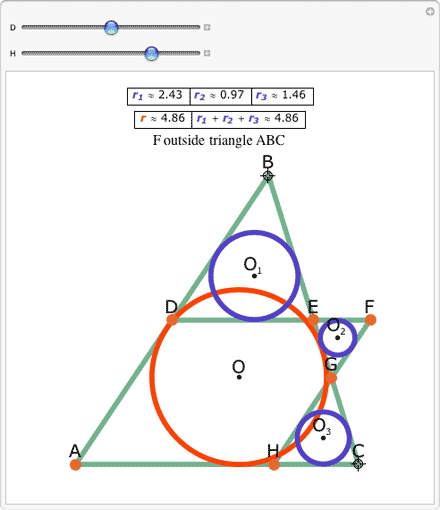With the picture in hand, the theorem is much simpler: the radii of the blue circles sum to the radius of the orange circle. One of the advantages of a Demonstration over a picture is that by moving the controls, we can see at a glance the range of situations covered by the theorem. The circles and triangles flex together in an intricate dance: complicated to describe, but easy and elegant to see. But beyond mere visual confirmation of what we already know to be true, this advantage extends to the power to easily consider situations not covered by the theorem. With the sliders adjusted so that F is inside the triangle ABC, the radius of the orange circle is no longer the sum of the radii of the blue circles, which is why the theorem stipulates that F is outside of ABC.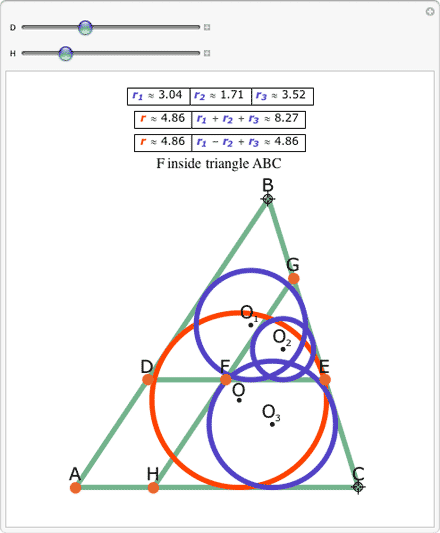Upon closer examination and some jiggling of the controls, it becomes clear that there is still a relationship among the four radii, however. It is now the case that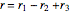. Thus the ability to quickly explore a wide range of mathematical possibilities visually leads to a generalization of the theorem. Whether F is inside or outside ABC, the triangle’s inradius (the radius of the orange circle) can be computed from the radii of the blue circles; it is just necessary to adjust the formula based on the location of F.

Jay Warendorff’s “The Radii of Four Incircles” Demonstration shows this more general version of the theorem. While I am sure this generalization is well known to advanced students of geometry, the web page that inspired this Demonstration gives only the one formula,. Demonstrations give us a chance not only to see and understand known theorems but also to glimpse stronger, more general theorems.